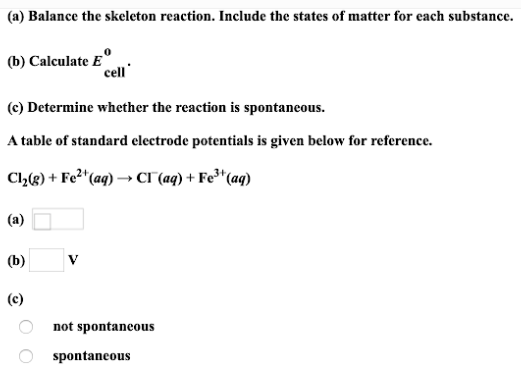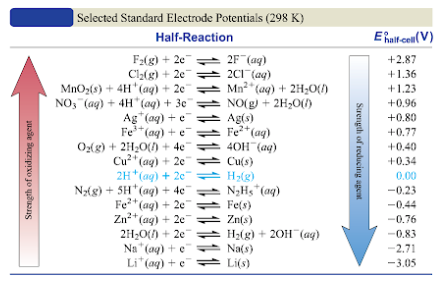# (a) Balanced the skeleton reaction. Include the states of matter for each substance. (b) Calculate E°cell. (c) Determine whether the reaction is spontaneous. A table of standard electrode potentials is given below for reference. Cl2(g) + Fe^2+(aq) → Cl^-(aq) + Fe^3+(aq)